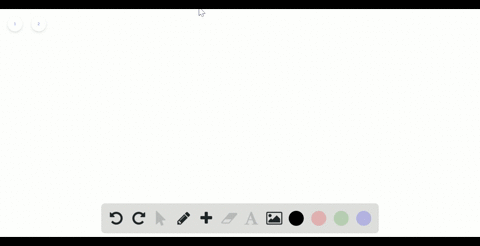Sign up for our free STEM online summer camps starting June 1st!View Summer Courses### The following diagram represents the collection o…

00:58Auburn University Main Campus

Need more help? Fill out this quick form to get professional live tutoring.

Get live tutoring
Problem 2

The following diagram shows the combination reaction between hydrogen, $\mathrm{H}_{2},$ and carbon monoxide, $\mathrm{CO},$ to produce methanol, $\mathrm{CH}_{3} \mathrm{OH}$ (white spheres are $\mathrm{H},$ black spheres are $\mathrm{C},$ red spheres are O). The correct number of CO molecules involved in this reaction is not shown. [ Section 3.1$]$
(a) Determine the number of CO molecules that should be shown in the left (reactants) box.
(b) Write a balanced chemical equation for the reaction.

a) four CO molecules
b) $4 \mathrm{CO}+8 \mathrm{H}_{2} \longrightarrow 4 \mathrm{CH}_{3} \mathrm{OH}$
c) $\mathrm{CO}+2 \mathrm{H}_{2} \longrightarrow \mathrm{CH}_{3} \mathrm{OH}$
d) See explanstion for solution.

## Discussion

You must be signed in to discuss.

## Video Transcript

Okay, So in this problem, we have to find out how many C o molecules should be placed here and here we can see. And the white fares shown here are high treason. That means we have a tight dress and molecule here in total. And we have four for me tunnel, which is produced from the reaction between carbon monoxide and hydrogen. So for me, tunnel is being produced here from the creation ofthe unknown number off carbon monoxide. And eight molik yourself hydrogen. So from here we have to find out how many molecules of carbon monoxide should be placed here. So to do that what we'LL do with first right down the reaction equation, which is carbon monoxide, will react to it. Hydrogen gas, the farm in this series three wage. So if we balance the equation first Well, you say that are so says we don't have any metal here. First, real balance or non metallic is carbon. So he had one carbon on its site. That this car was already balanced. Now a man is the head Rosen. We have to hundreds in here and four hydrogen in the product side. That means we have to multiply. This had risen by two tow have four hundred. And in the reactor site. Now we have one carbon and four hydrogen on the reactor inside and well, seeing the burdock site now we have to balance the oxygen and we can see that we have one oxygen on each side. That miss oxygen number is also balanced. This's the balance equation for the relation of carbon monoxide and hydrogen. Now that means one carbon monoxide will react to it. True hunters and monocle to form one molecule of them eternal. So if we have for me eternal proposed, that means we have to go multiply the reactor's side. Before also that missile we will require for carbon monoxide. And four times two, our eight had risen to have form eternal from this reaction. So and here you can. If you look at the diagram, we could see that we have for me eternal here. And eight hundreds in here. That man's we need four carbon monoxide toe complete this diagram, and in the diagram, there should be four carbon monoxide. Monica lt's in the reactor site and in the part you have to write down the balanced equation for destruction. And this's the balanced equation for carbon monoxide. Plus eight had risen equal toe four Ah, see three ways or maternal and ah, here is our balance situation for destruction.